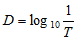# optical density

(redirected from Absorbance Unit)
Also found in: Dictionary, Thesaurus, Acronyms, Encyclopedia.

## optical density

The ability of a laboratory specimen to absorb or block the passage of light. The optical density of a laboratory sample can be used as an indicator of the concentration of specific components in the sample.
Synonym: optical absorbance

## optical density

a spectrophotometric measurement of light scattered by a suspension at a particular wavelength. Optical density measurements can be used to determine the BIOMASS concentration in a suspension, when, for instance, monitoring the GROWTH of a culture of MICROORGANISMS. It should be noted, however, that the microorganisms in the suspension will both scatter and, probably to a lesser extent, absorb the incident light, and it is the sum of these properties that is measured. It is therefore debatable what term should be applied. ABSORBANCE is sometimes used interchangeably with optical density, but this is not strictly correct. Optical density is generally accepted as the more appropriate term when measuring microbial growth.

## density, optical

A term applied to optical filters. It is equal to the logarithm to the base 10 of the reciprocal of the transmission factor T thus,where D is the symbol for optical density. Syn. absorbance. See absorbance; absorption; spectrophotometer; transmittance.
 Table D1 Density of optical lens materials n density (g/cm3) glass spectacle crown 1.523 2.54 dense barium crown 1.620 3.71 dense flint 1.706 3.20 dense barium flint 1.700 4.10 titanium oxide 1.701 2.99 plastics PMMA 1.490 1.19 CR-39 1.498 1.32 polycarbonate 1.586 1.2 cellulose acetate butyrate 1.48 1.2 cellulose propionate 1.46 1.2

 Table D2 Relationship between optical density D and light transmission T of optical filters D T (%) 0.0 100 0.1 79.4 0.2 63.1 0.3 50.1 0.4 39.8 0.5 31.6 0.6 25.1 0.7 20.0 0.8 15.8 0.9 12.6 1.0 10.0 1.5 3.16 2.0 1.0 2.5 0.32 3.0 0.1 4.0 0.01
References in periodicals archive ?
Caption: FIGURE 9: (a) Calculated absorbance unit by Beer-Lambert Law for A.
Measured, Mie, and CDA-predicted optically extinguished powers (E) were calculated as E = 1(1 - [10.sup.-A]), where A was 532 nm spectral extinction in absorbance units, based on measured, Mie, and CDA theory, respectively, and I was incident power of 532 nm irradiation (19-24 mW) for each AuNP-PDMS film.
EE6 calculates sensitivity two different ways: either by determining the lowest concentration significantly different from zero through use of instrument responses (e.g., absorbance units) of a zero and nonzero calibrator (analytical sensitivity) or by calculating the lowest concentration at which an analyte can be measured with acceptable precision (functional sensitivity).
Operating under a background absorbance of 0.02 au (absorbance units), a 5 x 10(sup 3)% pump-field variation causes greater thermal lens noise than what is contributed by the probe.
The uncertainties in the measurement of absorbance due to instrument noise were of the order of 0.001 absorbance units. There was an additional uncertainty of about 0.001 in suspension measurements due to the fluctuation of microsphere concentration.
(1) reported large CVs at or near the cutoff of 0.007 absorbance units (AU; 22% at 0.0062 AU and 17% at 0.007 AU); their calculations were based on manual drawing of a predicted baseline and measurement of net absorbance by use of a ruler.
For example, after 18 h, the absorbance was 0.041, 0.055, and 0.063 arbitrary absorbance units for Fe[Cl.sub.3], iron citrate, and Fe:NTA, respectively, at 32% theoretical [Fe.sup.3+] saturation.
A single NBA cut point of 0.007 absorbance units (AU) is recommended in the decision tree for interpretation and reporting of results (3).

Site: Follow: Share:
Open / Close